31.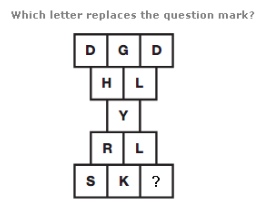A. J B. U C. Y D. E

Explanation: :

Working through the diagram in rows, from top to bottom, the sum of the numerical values of the letters in each row follows the sequence 15, 20, 25, 30, 35

32.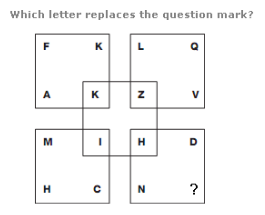A. V B. J C. I D. B

Explanation: :

Letters are arranged in each square in alphabetical order, skipping 5 letters at a time.

The letter bounded by the central square carries on this sequence, but using the reverse alphabetical value.

33.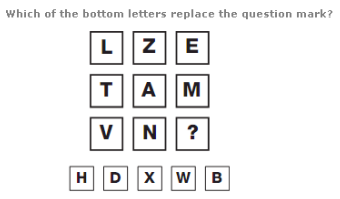A. N B. Y C. U D. W

Explanation: :

Looking at the diagram in columns, letters in the first column are written with 2 straight lines, in the second column they contain 3 straight lines, and letters in the right hand column are written with 4 straight lines.

34.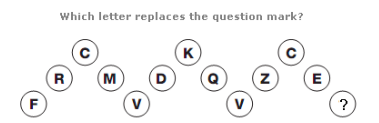A. Q B. T C. U D. F

Explanation: :

Moving from left to right, letters move though the alphabet in sequence, skipping 12 letters, then 11, 10, 9, 8 etc.

35.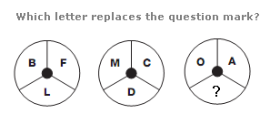A. N B. B C. J D. D